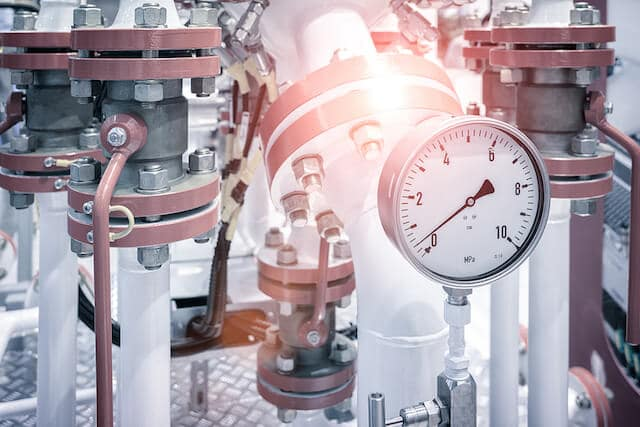Pressure

If you have ever hammered a nail or drank from a straw, you have made use of pressure to accomplish a task. A lot of the technology we use today work based on concepts of pressure or pressure differences, so there is no surprise that it is one of the most important topics in the study of physics.

Pressure is defined as the force acting normally per unit area. It has the following mathematical definition:

• Formula: Pressure = Force (N) / Area (m2)
• Simplified formula: P = F / a
• SI Unit: Pascal (Pa) or N/m2

Given this formula, we can immediately deduce a few characteristics of pressure. As the amount of force applied increases, the pressure also increases. However, as the area upon which the force is applied increases, pressure decreases. This is why pushing your finger against a wall will not make a dent, but hammering a nail’s sharp tip into the wall will puncture it with ease.

Transmission of pressure through liquids

Since liquids have mass, they can also be used to apply pressure on objects. Hydraulic systems take advantage of this fact by using an incompressible liquid to transmit pressure from a piston at one end of the system, to the piston at the other end. This works thanks to Pascal’s Principle, which states that pressure applied to a confined fluid is transmitted without loss to every part of the fluid and to the walls of the container.Source: Wikimedia Commons (edited)

Consider the diagram above. Since the pressure applied to the smaller piston (on the left) has to be equal to the pressure applied to the larger piston (on the right), you get the following formula:

• F1 / A1 = F2 / A2
• F1 = force applied to the smaller piston (N)
• F2 = force applied to the larger piston (N)
• A1 = area of the smaller piston (m2)
• A2 = area of the larger piston (m2)

Since the A1 is lower than A2, F2 will be higher than F1. In other words, the resultant force applied to the larger piston would be greater than the initial force applied to the smaller piston.

It is also important to note that the law of conservation of energy is at play here. As such, the work done on either piston must be equal. This is represented in the following formula:

• F1 x D1 = F2 x D2
• F1 = force applied to the smaller piston (N)
• F2 = force applied to the larger piston (N)
• D1 = distance travelled by the smaller piston (m)
• D2 = distance travelled by the larger piston (m)

Liquid pressure

Since liquids have mass, they can also exert pressure on objects. In a liquid column, liquid pressure is highly dependent on the height or depth of the liquid. At any given depth, the pressure experienced at any point is equal. This is because as the depth increases, the weight of the liquid above that point increases, applying more force. Furthermore, the pressure always acts perpendicular to the surface of the object.

Liquid pressure in a liquid column can be calculated using the following formula:

• Formula: Liquid pressure = Height of liquid (m) x Density of liquid (kg/m3) x gravitational field strength (N/kg)
• Simplified formula: P = hρg
• SI Unit: Pascal (Pa) or N/m2

Measuring atmospheric pressure

Atmospheric pressure is the pressure exerted by the weight of the air. At sea level, the average atmospheric pressure is defined as 101,325 Pa. However, it differs depending on several conditions. The most prominent condition is altitude, with atmospheric pressure dropping the higher up you go.Source: GCE Study Buddy

To conduct pressure measurement of atmospheric pressure, you can use a barometer (pictured above). A barometer consists of a liquid column, formed by a glass tube about 1m in length containing mercury. Above the mercury is a vacuum to allow for the mercury to rise and fall.

The weight (gravitational force) of the mercury and atmospheric pressure act simultaneously on the mercury. The mercury settles at a height where both of these forces balance each other out. The height of the mercury can then be used to calculate the atmospheric pressure using the following formula:

• Formula: Atmospheric pressure = Height of mercury (m) x Density of mercury (kg/m3) x gravitational field strength (N/kg)
• Simplified formula: P = hρg
• SI Unit: Pascal (Pa) or N/m2

Measuring gas pressure

Sometimes you will also need to measure the pressure of a gas. A manometer can be used for this purpose. It consists of a U-tube containing a liquid – typically mercury, oil, or water.Source: GCE Study Buddy

The horizontal portion of the manometer is connected to a gas supply, causing the liquid to be displaced. The resultant height of the liquid can be used to calculate the difference between the atmospheric pressure and pressure of the gas. The formula used is P = hρg, just like with the barometer (with the density changed to the liquid of choice).

• Formula: Pressure difference = Height of liquid (m) x Density of liquid (kg/m3) x gravitational field strength (N/kg)
• Simplified formula: P = hρg
• SI Unit: Pascal (Pa) or N/m2

Once the pressure difference is calculated, simply add the atmospheric pressure to it to find the pressure of the gas of interest.

Conclusion

There are many ways to conduct pressure measurements, and just as many ways to take advantage of pressure. Many of the formulas are similar, so you need to be vigilant to know when to use each one.

If you need additional help to understand the concepts related to pressure, or any other physics concepts, you can consider attending a physics tuition class. Here at Best Physics Tuition, our curriculum is designed to engage and excite young minds, while giving them the know-how they need to ace their physics exams.